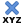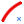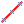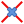# Points Panel

Use the Points panel to create free points using a wide variety of methods.

Location: Geom page

##XYZ Subpanel

Use the XYZ subpanel to create free points by specifying (x,y,z) coordinates.
There are several methods for entering the coordinate values.
• Manually specify the coordinate values in the x,y,z fields.
• Use the x,y,z selectors to select a node in the modeling window. The individual coordinate values are automatically populated in the corresponding fields.
• Use the as node selector to select a node in the modeling window. The individual x,y,z coordinate values and the reference system value from the selected node are automatically populated in the corresponding fields.

By default, free points are created relative to the global system; however, a local coordinate system can be specified using the system collector. This system can be rectangular, cylindrical or spherical. However, unlike nodes, free points cannot be assigned a reference system and are therefore not updated to reflect any subsequent changes made to the system.

##Arc Center Subpanel

Use the Arc Center subpanel to create free points at the center of the arc that best approximates the input set of nodes, points or lines.

For nodes and points input, a minimum of three must be selected.
For lines input, the selection must not all lie on a straight line.

An optional tolerance is available to verify whether the calculated best fit circle is sufficient. If ignored, the best fit circle is always calculated. Otherwise, an error is returned if the input entities are not all on the circle within the specified tolerance.

##Extract Parametric Subpanel

Use the Extract Parametric subpanel to create free points at parametric locations on lines and surfaces.

For lines:
• 0.0 <= u lower bound <= u upper bound <= 1.0.
• If the lower and upper bounds are the same, only one node is created.
• If the number of u nodes is specified as 1, only the lower bound is used.
• The line parameterization type can be specified as either arc length or internal.
For surfaces:
• 0.0 <= u lower bound <= u upper bound <= 1.0.
• 0.0 <= v lower bound <= v upper bound <= 1.0.
• The number of u and v free points must be >= 1.
• The total number of free points created equals (number of u points * number of v points).
• The surface parametric area is scaled to the visible surface area. Free points will be created inside the visible surface area.

##Intersect Subpanel

Use the Intersect subpanel to create free points at the intersection of geometric entities (lines/lines, lines/surfaces, lines/solids, lines/planes, vector/lines, vector/surfaces, vector/solids and vector/plane).

A free point is created at each intersection location.

For surface, solid and plane intersections, if the input line or vector lies on the surface/solid/plane the result is undefined and unpredictable.

For vectors, the magnitude and direction of the vector do not have any effect on the result. The vector extends through the entire model space.